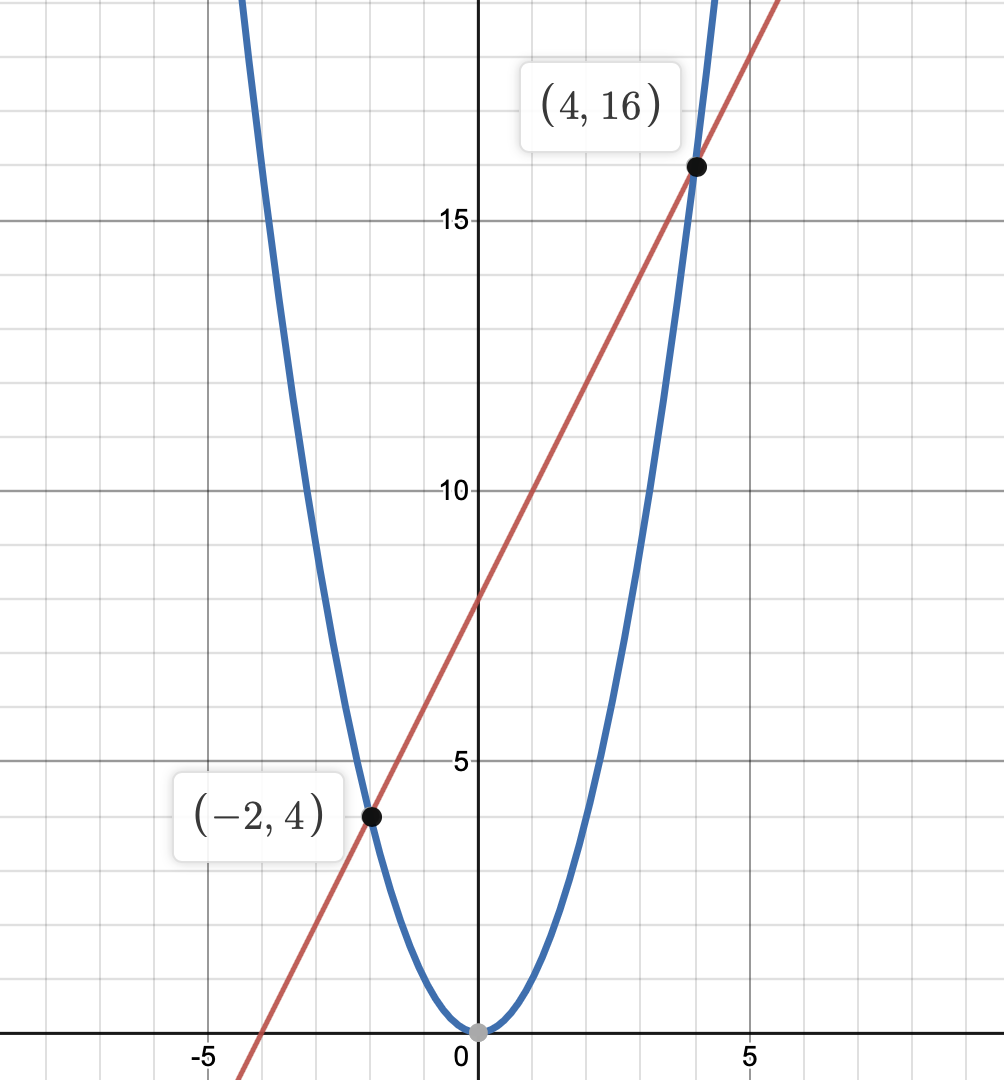# Week 2 – Calculus, Aggregation

Required:

Optional:

## Homework 2 (due Friday, January 21st at 11:59PM) (solutions) 📝

Submit your answers as a PDF to Gradescope by the due date for full credit. We encourage you to discuss the readings and questions with others in the course, but all work must be your own. Remember to use Campuswire if you need guidance!

### Question 1

Below in blue is the parabola $$y = x^2$$, and in red is the line $$y = 2x + 8$$.(a) Without using integration, determine the area between the given line and parabola.

This will involve a few steps. First, you’ll need to find the third point on the triangle that Archimedes specified in Quadrature of the Parabola. Some guiding questions:

• What is the slope of the red line?
• What is the slope of the tangent line to the parabola at any given point on the parabola? (What is the derivative of the parabola?)
• At what point on the parabola is the slope of the tangent line equal to the slope of the red line?

Once you’ve identified the third point, you can use this online calculator to find the area of that triangle (unless you’d rather do it yourself for practice). Then, you should be able to use a result from class. Show all of your steps, and remember to ask for help if you need it.

(b) If you’re familiar with integration, compute the area between the given line and parabola using integration, and show that your answer is the same as your answer in part (a).

(Note: If you know how to take an integral, you are expected to do this question.)

### Question 2

Consider the function

$f(x) = x(48 - x^2)$

(a) Using Fermat’s method of adequality, determine the value of $$x$$ that maximizes $$f(x)$$. Is this a local or global maximum? Show your work.

(b) Using Fermat’s method of adequality, determine the slope of the tangent line to $$f(x)$$ at $$x = 1$$. Verify that your answer is correct by finding $$f'(x)$$ and evaluating $$f'(1)$$. Show your work.

(c) Repeat part (b), but at $$x = 0$$. What issue do you run into?

### Question 3

Show that Fermat’s method for finding the slope of the tangent line is equivalent to

$\text{slope} = \frac{f(x+e) - f(x)}{e}$

Does the quotient on the right look familiar – if so, what does it resemble?

Hopefully, by answering this question, it is slightly more clear why Fermat’s method of adequality involves dividing by $$e$$ and then setting $$e$$ to 0.

### Question 4

One aggregation strategy that was used centuries ago involved picking the number halfway between the smallest and largest numbers in a dataset. In today’s terminology, we’d call this number the “midrange”.

$\text{Midrange} = \frac{\text{Max}(x) + \text{Min}(x)}{2}$

For each of the following conditions, specify a set of 5 unique numbers that satisfies the given condition. In each case, compute the midrange and mean and show that the condition holds. Afterwards, give a one sentence answer to the question, “for what kinds of datasets is the midrange close to the mean?”

(a) Midrange = mean

(b) Midrange > mean

(c) Midrange < mean